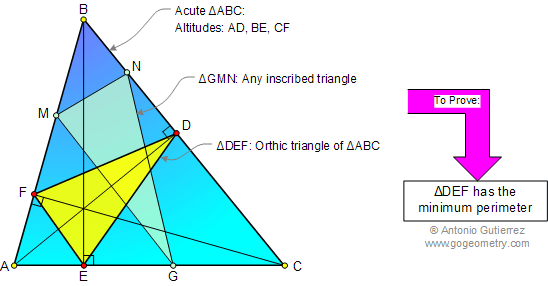# Geometry Problem 51 with Solution: Fagnano's Triangle

< PREVIOUS PROBLEM  |  NEXT PROBLEM >

In a given acute triangle ABC, prove that the inscribed triangle with the minimum perimeter is the orthic triangle DEF.

Orthic triangle: The triangle whose vertices are the feet of the altitudes of a given triangle.Hints: See Geometry Help

Home | Geometry | Altitude | Problems | Open Problems | 10 Problems | 51-60 | Semiperimeter | Orthic Triangle | Email | by Antonio Gutierrez